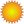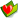cht 電腦資訊 文書排版 TeX TeX Tips# 使國字帶有注音符號(chitex)

yih
joined: 2008-12-03
posted: 12
promoted: 3
bookmarked: 3
 1 subject: 使國字帶有注音符號(chitex)11 2008-12-11 quote

http://blog.bs2.to/post/EdwardLee/6091

\documentclass[12pt]{article}
\usepackage{graphicx}

\begin{document}

\makeatletter
\newskip\ch@dimtmpb
\newskip\ch@dimtmpc
\newskip\ch@dimtmpd
\newskip\ch@dimtmpe
\newskip\ch@dimtmpf
\newskip\ch@nraise

\newbox\ch@nboxa
\newbox\ch@nboxb

\def\chcheck#1{%
\setbox\ch@nboxa\hbox{ㄑ}%
\setbox\ch@nboxb\hbox{#1}%
\ch@dimtmpb=\wd\ch@nboxa%
\ch@dimtmpc=\wd\ch@nboxa%
\ch@dimtmpd=\wd\ch@nboxa%
\divide\ch@dimtmpb by 2%
\multiply\ch@dimtmpc by 3%
\multiply\ch@dimtmpd by 2%
\ifdim\ch@dimtmpe < 0pt \multiply \ch@dimtmpe by -1 \fi%
\ifdim\ch@dimtmpf < 0pt \multiply \ch@dimtmpf by -1 \fi%
\ifdim\ch@dimtmpe < \ch@dimtmpb%
\def\ch@chkount{3}%
\else%
\ifdim\ch@dimtmpf > \ch@dimtmpb%
\def\ch@chkount{1}%
\else%
\def\ch@chkount{2}%
\fi%
\fi}

\def\ch@koa{1}
\def\ch@kob{2}
\def\ch@koc{3}

\def\chsetmag#1{\ch@fntdimtmpa=\ch@fntdimone%
\multiply\ch@fntdimone by #1\divide\ch@fntdimone by 1000}%

\def\BPM#1#2#3{%
\chcheck{#2}%
\def\ch@ntmp{}\def\ch@ttmp{#3}%
#1%
\ifx\ch@chkount\ch@koc%%
\ch@nraise=1.85ex
\else%
\ifx\ch@chkount\ch@kob%
\ch@nraise=1.6ex%
\else%
\ch@nraise=1.05ex%
\fi%
\fi%
{\uming\chsetmag{340}\raisebox{\ch@nraise}{%
\rotatebox{-90}{{\chvertical #2}}}}%
\ifx\ch@ttmp\ch@ntmp\else%
{\uming%
\chsetmag{340}\hspace*{-.25ex}\raisebox{0.2ex}{#3}}\hspace*{-.25ex}\mbox{}%
\fi%
}

{\ukai
\BPM{全}{ㄑㄩㄢ}{ˊ}\BPM{注}{ㄓㄨ}{ˋ}\BPM{音}{ㄧㄣ}{}%
\BPM{一}{ㄧ}{}
}

{\uming
\BPM{全}{ㄑㄩㄢ}{ˊ}\BPM{注}{ㄓㄨ}{ˋ}\BPM{音}{ㄧㄣ}{}%
\BPM{一}{ㄧ}{}
}

{\uming\LARGE
\BPM{全}{ㄑㄩㄢ}{ˊ}\BPM{注}{ㄓㄨ}{ˋ}\BPM{音}{ㄧㄣ}{}%
\BPM{一}{ㄧ}{}
}

\end{document}

Hung-Yih Chen

yih
joined: 2008-12-03
posted: 12
promoted: 3
bookmarked: 3
 2 subject: 使國字帶有注音符號(chitex)00 2008-12-13 quote
 yih 在     http://blog.bs2.to/post/EdwardLee/6091 中, Edward 曾提出有關注音符號排版的作法， 在 chitex 有另外的方法, 可以不受中文字型的不同與大小的影響 ..................................... \def\BPM#1#2#3{% .................................... {\uming\LARGE \BPM{全}{ㄑㄩㄢ}{ˊ}\BPM{注}{ㄓㄨ}{ˋ}\BPM{音}{ㄧㄣ}{}% \BPM{一}{ㄧ}{} }

在上面之方法對於含二字元之注音的顯示不是很理想, 我做了一些小修改

看來好些, 如下:

\documentclass[12pt]{article}
\usepackage{graphicx}

\begin{document}

\makeatletter
\newskip\ch@dimtmpb
\newskip\ch@dimtmpc
\newskip\ch@dimtmpd
\newskip\ch@dimtmpe
\newskip\ch@dimtmpf
\newskip\ch@nraise
\newbox\ch@nboxa
\newbox\ch@nboxb

\def\chcheck#1{%
\setbox\ch@nboxa\hbox{ㄑ}%
\setbox\ch@nboxb\hbox{#1}%
\ch@dimtmpb=\wd\ch@nboxa%
\ch@dimtmpc=\wd\ch@nboxa%
\ch@dimtmpd=\wd\ch@nboxa%
\divide\ch@dimtmpb by 2%
\multiply\ch@dimtmpc by 3%
\multiply\ch@dimtmpd by 2%
\ifdim\ch@dimtmpe < 0pt \multiply \ch@dimtmpe by -1 \fi%
\ifdim\ch@dimtmpf < 0pt \multiply \ch@dimtmpf by -1 \fi%
\ifdim\ch@dimtmpe < \ch@dimtmpb%
\def\ch@chkount{3}%
\else%
\ifdim\ch@dimtmpf > \ch@dimtmpb%
\def\ch@chkount{1}%
\else%
\def\ch@chkount{2}%
\fi%
\fi}

\def\ch@koa{1}
\def\ch@kob{2}
\def\ch@koc{3}

\def\chsetmag#1{\ch@fntdimtmpa=\ch@fntdimone%
\multiply\ch@fntdimone by #1\divide\ch@fntdimone by 1000}%
\def\ch@gettwo#1#2#3#4{\def\ch@one{#1#2}\def\ch@two{#3#4}} % 新增

\def\BPM#1#2#3{%
\chcheck{#2}%
\def\ch@ntmp{}\def\ch@ttmp{#3}%
#1%
\ifx\ch@chkount\ch@koc%%
\ch@nraise=1.85ex
\else%
\ifx\ch@chkount\ch@kob%
\ch@nraise=1.6ex%
\ch@gettwo #2\relax%
\else%
\ch@nraise=1.05ex%
\fi%
\fi%
\ifx\ch@chkount\ch@kob%                                         % 新增
{\uming\chsetmag{340}%                                         % 新增
\setbox\ch@nboxa\hbox{ㄑ}%                                   % 新增
\ch@dimtmpb=\wd\ch@nboxa%                                  % 新增
\ch@dimtmpc=\wd\ch@nboxb%                                   % 新增
\multiply\ch@dimtmpb by 2\divide\ch@dimtmpb by 5%   % 新增
\setbox\ch@nboxb\hbox{\rotatebox{-90}{\ch@one}}%  % 新增
\ch@dimtmpc=\wd\ch@nboxb%                                    % 新增
\divide\ch@dimtmpc by 6%                                           % 新增
\raisebox{\ch@nraise}{%                                             % 新增
\rotatebox{-90}{\hspace*{-\ch@dimtmpc}{\chvertical\ch@one}\hspace{\ch@dimtmpb}{\chvertical\ch@two}}}}% % 新增
\else%                                                                         % 新增
{\uming\chsetmag{340}\raisebox{\ch@nraise}{%
\rotatebox{-90}{{\chvertical #2}}}}%
\fi                                                                                 % 新增
\ifx\ch@ttmp\ch@ntmp%
\hspace{.175ex}%
\else%
{\uming%
\chsetmag{340}\hspace*{-.25ex}\raisebox{0.2ex}{#3}}\hspace*{-.25ex}\mbox{}%
\fi\hspace{.1ex}%
}

{\ukai
\BPM{全}{ㄑㄩㄢ}{ˊ}\BPM{注}{ㄓㄨ}{ˋ}\BPM{音}{ㄧㄣ}{}%
\BPM{一}{ㄧ}{}
}

{\uming
\BPM{全}{ㄑㄩㄢ}{ˊ}\BPM{注}{ㄓㄨ}{ˋ}\BPM{音}{ㄧㄣ}{}%
\BPM{一}{ㄧ}{}
}

{\uming\LARGE
\BPM{全}{ㄑㄩㄢ}{ˊ}\BPM{注}{ㄓㄨ}{ˋ}\BPM{音}{ㄧㄣ}{}%
\BPM{一}{ㄧ}{}
}

\end{document}

[img]#1[/img]

yih
joined: 2008-12-03
posted: 12
promoted: 3
bookmarked: 3
 3 subject: 使國字帶有注音符號(utf-8 及 非 chitex)00 2008-12-23 quote
 yih 在上面之方法對於含二字元之注音的顯示不是很理想, 我做了一些小修改 --------------------------------- \makeatletter \newskip\ch@dimtmpb \newskip\ch@dimtmpc -------------------------------- \def\ch@gettwo#1#2#3#4{\def\ch@one{#1#2}\def\ch@two{#3#4}} % 新增 ---------------

\def\ch@gettwo#1#2#3#4#5#6{\def\ch@one{#1#2#3}\def\ch@two{#4#5#6}}

edited: 1
yih
joined: 2008-12-03
posted: 12
promoted: 3
bookmarked: 3
 4 subject: 使國字帶有注音符號(utf-8 及 非 chitex)00 2008-12-23 quote
 yih 若為 utf-8 內碼檔   須稍加修改. ........

macros 無法使用, 因其中用到  chitex 中字型大小的 \ch@fntdimone

yih
joined: 2008-12-03
posted: 12
promoted: 3
bookmarked: 3
 5 subject: 使國字帶有注音符號(utf-8 及 非 chitex)00 2008-12-24 quote
 yih 很抱歉, 在原來的第三篇 (現已修改) 提供的 "不是 chitex 上" 的使國字帶有注音符號的 macros 無法使用, 因其中用到  chitex 中字型大小的 \ch@fntdimone  原來的第三篇 現已修改, 只有在 chitex 中 utf-8 內碼檔時 macros 的 modify

\documentclass[12pt]{article}
\usepackage{graphicx}

\begin{document}

\makeatletter % 若為 Utf-8 去掉下列3-4行前之% 且將1-2 行mark

\def\chgettwo#1#2#3#4{\def\ch@one{#1#2}\def\ch@two{#3#4}}
\def\chgetthr#1#2#3#4#5#6{\def\ch@one{#1#2}\def\ch@two{#3#4}\def\ch@thr{#5#6}}
%\def\chgettwo#1#2#3#4#5#6{\def\ch@one{#1#2#3}\def\ch@two{#4#5#6}}
%\def\chgetthr#1#2#3#4#5#6#7#8#9{\def\ch@one{#1#2#3}\def\ch@two{#4#5#6}\def\ch@thr{#7#8#9}}
\def\ch@va#1{\hspace*{.2ex}\rotatebox{90}{#1}}
\def\ch@vb#1{\chgettwo#1%
\hspace*{.2ex}\rotatebox{90}{\ch@one}\rotatebox{90}{\ch@two}}
\def\ch@vc#1{\chgetthr#1%
\hspace*{.35ex}\rotatebox{90}{\ch@one}\rotatebox{90}{\ch@two}\rotatebox{90}{\ch@thr}}

\newskip\ch@dimtmpb
\newskip\ch@dimtmpc
\newskip\ch@dimtmpd
\newskip\ch@dimtmpe
\newskip\ch@dimtmpf
\newskip\ch@nraise
\newbox\ch@nboxa
\newbox\ch@nboxb

\def\chcheck#1{%
\setbox\ch@nboxa\hbox{ㄑ}%
\setbox\ch@nboxb\hbox{#1}%
\ch@dimtmpb=\wd\ch@nboxa%
\ch@dimtmpc=\wd\ch@nboxa%
\ch@dimtmpd=\wd\ch@nboxa%
\divide\ch@dimtmpb by 2%
\multiply\ch@dimtmpc by 3%
\multiply\ch@dimtmpd by 2%
\ifdim\ch@dimtmpe < 0pt \multiply \ch@dimtmpe by -1 \fi%
\ifdim\ch@dimtmpf < 0pt \multiply \ch@dimtmpf by -1 \fi%
\ifdim\ch@dimtmpe < \ch@dimtmpb%
\def\ch@chkount{3}%
\else%
\ifdim\ch@dimtmpf > \ch@dimtmpb%
\def\ch@chkount{1}%
\else%
\def\ch@chkount{2}%
\fi%
\fi}

\def\ch@koa{1}
\def\ch@kob{2}
\def\ch@koc{3}

\def\BPM#1#2#3{%
\chcheck{#2}%
\def\ch@ntmp{}\def\ch@ttmp{#3}%
#1%
\ifx\ch@chkount\ch@koc%%
\let\chvert\ch@vc
\ch@nraise=1.85ex
\else%
\ifx\ch@chkount\ch@kob%
\let\chvert\ch@vb
\ch@nraise=1.6ex%
%\ch@gettwo #2\relax%
\chgettwo #2\relax%
\else%
\let\chvert\ch@va%
\ch@nraise=1.05ex%
\fi%
\fi%
\ifx\ch@chkount\ch@kob%
{\mingu\fontsize{0.78967791ex}{0.19354826ex}%
\setbox\ch@nboxa\hbox{ㄑ}%
\ch@dimtmpb=\wd\ch@nboxa%
\ch@dimtmpc=\wd\ch@nboxb%
\multiply\ch@dimtmpb by 2\divide\ch@dimtmpb by 5%
\setbox\ch@nboxb\hbox{\rotatebox{-90}{\ch@one}}%
\ch@dimtmpc=\wd\ch@nboxb%
\divide\ch@dimtmpc by 6%
\raisebox{\ch@nraise}{%
\rotatebox{-90}{\hspace*{-\ch@dimtmpc}{\ch@va{\ch@one}}\hspace{\ch@dimtmpb}{\ch@va{\ch@two}}}}}%
\else%
{\mingu\fontsize{0.78967791ex}{0.19354826ex}\raisebox{\ch@nraise}{%
\rotatebox{-90}{{\chvert{#2}}}}}%
\fi
\ifx\ch@ttmp\ch@ntmp%
\hspace{.175ex}%
\else%
{\mingu%
\fontsize{0.78967791ex}{0.19354826ex}\hspace*{-.25ex}\raisebox{0.2ex}{#3}}\hspace*{-.25ex}\mbox{}%
\fi\hspace{.1ex}%
}

{\kaiu
\BPM{全}{ㄑㄩㄢ}{ˊ}\BPM{注}{ㄓㄨ}{ˋ}\BPM{音}{ㄧㄣ}{}%
\BPM{一}{ㄧ}{}
}

{\mingu
\BPM{全}{ㄑㄩㄢ}{ˊ}\BPM{注}{ㄓㄨ}{ˋ}\BPM{音}{ㄧㄣ}{}%
\BPM{一}{ㄧ}{}
}

{\mingu\LARGE
\BPM{全}{ㄑㄩㄢ}{ˊ}\BPM{注}{ㄓㄨ}{ˋ}\BPM{音}{ㄧㄣ}{}%
\BPM{一}{ㄧ}{}
}

\end{document}

edited: 1

joined: 2008-04-01
posted: 185
promoted: 20
bookmarked: 7
 6 subject:00 2008-12-25 quote

yih
joined: 2008-12-03
posted: 12
promoted: 3
bookmarked: 3
 7 subject:00 2008-12-26 quote
 企鵝狂 我沒有在文件裡面用過注音 不過想問一下,如果從像是輸入法的table裡面,找出不是破音字的字,是不是就可以半自動幫文件加上注音了? 比較複雜的字甚至可以用到像是詞音的辭典之類的。 不知道這個想法可不可行...有空的話蠻想來試試看的...

寫一個 prepocessor 應該可以cht 電腦資訊 文書排版 TeX TeX Tips